﻿ 黑龙江科技大学学报

(1.黑龙江科技大学 教学质量评估中心, 哈尔滨 150022; 2.黑龙江科技大学 机械工程学院, 哈尔滨 150022)

Optimization design of planetary mechanism of shearer cutting part based on fireworks algorithm
Wu Weidong1, Ma Yang2, Cai Shuwen2, Zhou Xingping2, Xu Wei2
(1.Teaching Quality Evaluation Center, Heilongjiang University of Science & Technology, Harbin 150022,China; 2.School of Mechanical Engineering, Heilongjiang University of Science & Technology, Harbin 150022, China)
This paper seeks to improve the bearing capacity of the final planetary mechanism of the cutting section of the shearer. The improvement is achieved by developing an optimization model with the bending stress and total volume of planetary gear as objective functions by selecting as design variables 7 main parameters, such as gear, module and displacement coefficient, under 11 inequality constraints such as transmission ratio and overlap degree constraints and 3 equation constraints. The study includes solving the problem by considering the multi-peak characteristics of the planetary mechanism optimization model and using the spark algorithm; constructing the adaptive explosion radius and learning operator by introducing the Sigmoid function to improve the spark algorithm; and performing standard tests and offset tests using six typical test functions. The results show that the improved firework algorithm features a better optimization ability and robustness; and the optimization is followed by a 0.95% reduction in the total volume, with a 15.9%, 15.8% and 16.7% reduction respectively for the bending stress of solar wheel(302 MPa), the bending stress of planetary wheel(299 MPa), and the bending stress of inner ring gear(224 MPa).

1 数学模型的建立1.1 设计变量的选取

X=(za,zc,zb,m,xa,xb,xc)T

1.2 目标函数的确定

σF=(Ft)/(bm)YFaYSaYεYβKAKVKK,

KV——动载系数,文中取1.035;

K——齿向载荷分布系数,文中取1.204;

K——齿间载荷分布系数,文中取1;

Yε——弯曲强度重合度系数,Yε=0.25+0.75/ε;

ε——对应齿轮对的重合度;

YFa——齿形系数;

YSa——应力修正系数;

Yβ——螺旋角系数,文中取1。

f1(x)=(519 120)/(zabm2)(0.25+0.75/εac),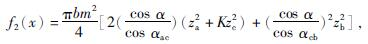αac、αcb——对应轮组啮合角。

F(x)=w1 fc f1(x)+w2 fv f2(x),

w1(t)=|sin(2πt/f)|,

w2(t)=1-w1(t)。

1.3 约束条件的确定

(1)传动比约束

g1=|(1+zb/za)-5.06|-0.5≤0。

(2)重合度约束

g2=1.2-1/(2π)[za(tan αa-tan αac)+ zc(tan αc-tan αac)]≤0,

g3=1.2-1/(2π)[zc(tan αc-tan αcb)- zb(tan αb-tan αcb)]≤0。

(3)齿顶厚约束

g4=0.25m-(mza+2m(1+xa-Δy))·((π)/(2z)a+(2xa)/(za)tan α+inv α-inv αa)≤0,

g5=0.25m-(mzc+2m(1+xc-Δy))·((π)/(2zc)+(2xc)/(zc)tan α+inv α-inv αc)≤0,

g6=0.25m-(mzb-2m(1-xb-Δy))·((π)/(2zb)-(2xb)/(zb)tan α-inv α+inv αb)≤0,

(4)齿轮过渡曲线干涉约束

g7=(zc)/(za)(tan αc-tan αac)-tan αac+tan α-(4(1-xa))/(zasin 2α)≤0,

g8=(za)/(zc)(tan αa-tan αac-tan αac+tanα-(4(1-xc))/(zcsin 2α)≤0。

(5)齿面接触强度约束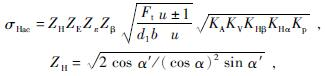Zε——重合度系数,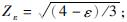ZE——弹性系数,文中取值为189.812 N/MPa;

Zβ——螺旋角系数,文中取1;

u——齿数比。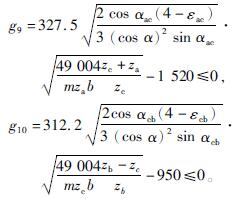(6)行星齿轮的领接条件约束

g11=zc+2(1+xc)-(za+zc)sin(π)/4<0。

(7)齿轮的无侧隙啮合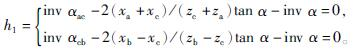(8)行星轮系同心条件

h2=(za+zc)/(cos αac)-(zb-zc)/(cos αcb)=0。

(9)装配条件

h3=(za+zc)/4=C,

2 改进的烟花算法2.1 烟花算法

FWA以模拟烟花爆炸作为随机探索机制,在选择策略中平衡适应度在与个体在种群中聚集情况,使烟花算法构建一种平衡局部探索和全局探索资源分配的方式,各算子具体运行方式如下。

(1)种群的初始化

xi=(xmax-xmin)rand(1,D)+xmin,

D——变量维度;

xij——第i个烟花j个维度上的分量。

(2)爆炸火花的范围和数量fmax、fmin——f(xi)的适应度最大值、最小值;

e——定义的极小量。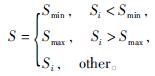(3)子代火花生成

xik=xik+hk,

hk=Airand(-1,1)。

(4)高斯变异火花

xkj=xkiGaussian(1,1)。

xjk=xmink+rand(0,1)(xkmax-xkmin)。

(5)迭代选择方式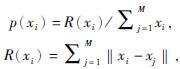2.2 改进策略

Fig.1 Flow of improved fireworks algorithm

(1)自适应爆炸范围

at=1/(1+et/T·n1)+n2,

T——总迭代次数;

t——当前迭代次数。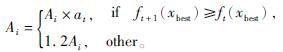(2)学习算子

Δx=xi+cφ(k)(gbest(xi)-xi),

c——自定义学习因子。

3 算法仿真测试3.1 标准函数的测试

Fig.2 Function test curve求解50次,得到最优数值解、平均解和方差统计见表1

Table 1 Optimization results of standard test function

3.2 偏移函数测试

Table 2 Offset index and offset

Fig.3 Offset function test curve

Table 3 Experiment of different migration index for FWA

Table 4 Experiment of different migration index for AFWA

4 优化模型的求解

Fig.4 Pareto front of volume and wheel bending stress

Table 5 Comparison between optimization results

Table 6 Comparison between optimization results

5 结 论

(1)通过Sigmoid自适应函数和学习算子,有效提高烟花算法的寻优性能并和稳健性。实验结果证明,AFWA在6种测试函数上的寻优性能与传统烟花算法相比是有着明显的优势。

(2)应用改进后的算法优化行星机构多目标,并设置动态权重分配,使得目标权重分配合理并获得较好pareto前沿,总体积降低0.95%,太阳轮弯曲应力减小15.9%、内齿圈弯曲应力减小16.7%,行星轮弯曲应力减小15.8%,优化后齿轮承载能力显著提高。